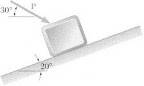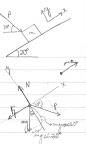# Finding the force of a block

• vipertongn
In summary: The 301 N answer for motion seems to check out though.In summary, the problem involves a 20-kg package on an incline with a force P being applied to it. The magnitude of P is to be determined, given that it takes 10 seconds for the package to travel 5m up the incline and the coefficients of friction are both 0.3. The sum of forces equations are set up in the x- and y-directions, with the angle of P being 50 degrees from the horizontal. The equation for P is then solved, resulting in a value of 301 N for motion and an unclear value for the first answer.

## Homework Statement

A 20-kg package is at rest on an incline when a force P is applied to it. Determine the magnitude of P if 10s is required for the package to travel 5m up the incline. The static and kinetic coefficients of friction between the package and the incline are both equal to 0.3.Answer: 419N to start and 301 during sliding

## Homework Equations

m=20kg
g=9.8m/s2
t=10s
xi = 0ft
xf = 5ft
μs = μk = 0.3
Frictional force = Ff = μN

## The Attempt at a Solution

First, I drew a free-body diagram including tilted coordinate axes:Then, I went about finding the sum of the forces equations in the x- and y-directions.

$$\sum F_{x}=-F_{f}-mgsin(20)+Pcos(30)=ma$$

$$\sum F_{y}=N-mgcos(20)-Psin(30)=0$$

From the second equation,
$$N=mgcos(20)+Psin(30)$$

Plugging this value for N into Ff = μN in the first equation yields:

$$P=\frac{ma+\left(\mg(mu cos(20)+sin(20)\right)}{cos(30)-\mu sin(30)}$$

My problem is that I know for impending motion you set a= 0 so plugged in I get
P=296 N
and for motion I get
P=301

Problem is the first P is suppose to be 419 and I don't know why I'm not getting the right answer.

I don't think you have made the direction of P clear.
The angle is not mentioned at all in the question.
In your upper diagram, it is shown 30 degrees below horizontal.
In your lower diagram, it is shown 30 degrees below the angle of the ramp.
Can't be both.

vipertongn said:
Then, I went about finding the sum of the forces equations in the x- and y-directions.

$$\sum F_{x}=-F_{f}-mg \sin(20)+P \cos({\color{red}30})=ma$$

$$\sum F_{y}=N-mg \cos(20)-P \sin({\color {red}30})=0$$

From the second equation,
$$N=mg \cos(20)+P \sin({\color{red}30})$$
(Red emphasis mine. Also made a slight modification to the "sin" and "cos" notation in LaTeX.)

The force P is 30o with respect to the horizontal But your definition of the x-axis is not the horizontal. What angle is P with respect to your definition of the the x-axis?

[Edit: Delphi51 beat me to the hint.]

ohh it was drawn wrong...ok the new angle for P is 50 degrees from the horizontal of my new axis. I made my new equation to be
P= ma+mg(sin20+ mu cos20)
-----------------------------------------------
cos50- mu sin 50

I didn't get the same thing. Doesn't mean you're wrong, but it would be nice to synchronize our work.
For normal force, I have Fn = mg*cos(20) + P*sin(40).
Do you agree?

I'm not sure about that, i used 50 degrees because i set the x-axis to be the same as the block, and then used the transversal angles are equal...so adding 20 to 30 degrees to get that. It's ironic because when I solved for the motion...it came to the correct answer of 301, but for the first answer it doesn't work...and the only difference is adding 2 (ma) to the numerator

You could write it as cos(50) instead of sin(40).
Fn = mg*cos(20) + P*cos(50)

yea but the overall equation still doesn't make out to be 419 as shown in the solutions

vipertongn said:
yea but the overall equation still doesn't make out to be 419 as shown in the solutions
Are you sure that the static coefficient of friction is also 0.3? Is everything related to the problem given in the problem statement (nothing left out)? If so, I think there might be some sort of mistake in the given solution regarding the "419 N to start" answer.